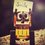# Domain help

What is domain of $\sqrt{[x]-2x}$

$[•]$ represents greatest integer function.

May sound silly question but I have just started learning functions, so plz help.Note by Kushal Patankar
6 years, 2 months ago

This discussion board is a place to discuss our Daily Challenges and the math and science related to those challenges. Explanations are more than just a solution — they should explain the steps and thinking strategies that you used to obtain the solution. Comments should further the discussion of math and science.

When posting on Brilliant:

• Use the emojis to react to an explanation, whether you're congratulating a job well done , or just really confused .
• Ask specific questions about the challenge or the steps in somebody's explanation. Well-posed questions can add a lot to the discussion, but posting "I don't understand!" doesn't help anyone.
• Try to contribute something new to the discussion, whether it is an extension, generalization or other idea related to the challenge.
• Stay on topic — we're all here to learn more about math and science, not to hear about your favorite get-rich-quick scheme or current world events.

MarkdownAppears as
*italics* or _italics_ italics
**bold** or __bold__ bold
- bulleted- list
• bulleted
• list
1. numbered2. list
1. numbered
2. list
Note: you must add a full line of space before and after lists for them to show up correctly
paragraph 1paragraph 2

paragraph 1

paragraph 2

[example link](https://brilliant.org)example link
> This is a quote
This is a quote
    # I indented these lines
# 4 spaces, and now they show
# up as a code block.

print "hello world"
# I indented these lines
# 4 spaces, and now they show
# up as a code block.

print "hello world"
MathAppears as
Remember to wrap math in $$ ... $$ or $ ... $ to ensure proper formatting.
2 \times 3 $2 \times 3$
2^{34} $2^{34}$
a_{i-1} $a_{i-1}$
\frac{2}{3} $\frac{2}{3}$
\sqrt{2} $\sqrt{2}$
\sum_{i=1}^3 $\sum_{i=1}^3$
\sin \theta $\sin \theta$
\boxed{123} $\boxed{123}$

## Comments

Sort by:

Top Newest

Could you please clarify what $[x]$ means; is it the greatest integer function?

- 6 years, 2 months ago

Log in to reply

Updated

- 6 years, 2 months ago

Log in to reply

O.k., great. Assuming that we are working in the domain of real numbers, we require that $g(x) = [x] - 2x \ge 0.$

Now clearly for $x \gt 0$ we have $g(x) \lt 0$ since it can be at most $x - 2x = -x.$ Since $g(0) = 0$ we have that $0$ is part of the domain of $f(x) = \sqrt{g(x)}.$

Next, for $-\frac{1}{2} \lt x \lt 0$ we have that $g(x) = -1 - 2x \lt 0,$ and hence this interval is not part of the domain of $f(x).$ However, for $-1 \le x \le -\frac{1}{2}$ we have that $g(x) = -1 - 2x \ge 0.$

For $-2 \le x \le -1$ we have that $g(x) = -2 - 2x = -2(1 + x) \ge 0$ since $1 + x \le 0.$

In general, for $k \le x \le (k + 1)$ for integers $k \le -2$ we see that $g(x) = k - 2x \ge k - 2(k + 1) = -(k + 2) \ge 0.$

So putting the pieces together, we conclude that the domain of $f(x)$ is $(-\infty, -\frac{1}{2}] \cup \{0\}.$

Edit: I just checked WolframAlpha; oddly, it responded with "unable to determine domain". I think I've got it right, though; it's an unusual, discontinuous function, but breaking down the intervals as I have reveals the general form of the function.

- 6 years, 2 months ago

Log in to reply

I am getting the same.

- 6 years, 2 months ago

Log in to reply

Great. Thanks for the confirmation. :)

- 6 years, 2 months ago

Log in to reply

Thanks for the answer. But I didn't got the reason behind taking $x \in (\frac{-1}{2} , 0)$ as a case.

- 6 years, 2 months ago

Log in to reply

In that interval we have $g(x) = -1 - 2x \lt -1 - 2(\frac{1}{2}) = 0.$ Thus $f(x) = \sqrt{g(x)}$ is not defined (over the reals) in that interval. In the interval $[-1, -\frac{1}{2}]$ we have $g(x) = -1 - 2x \ge -1 - 2(\frac{1}{2}) = 0,$ and so $f(x)$ is defined in this interval. This is why we had to isolate the interval $(-\frac{1}{2}, 0).$

- 6 years, 2 months ago

Log in to reply

OK. got you now. Thanks 4 help

- 6 years, 2 months ago

Log in to reply

You're welcome. :)

- 6 years, 2 months ago

Log in to reply

We could easily visualise it graphically.

- 5 years, 1 month ago

Log in to reply

×

Problem Loading...

Note Loading...

Set Loading...